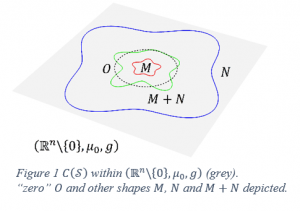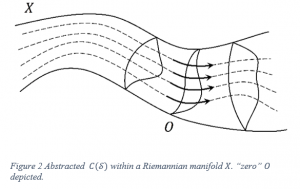The original title for this project was “Subsets of the powerset of R^n as inner product spaces”. Meaning I wanted to find (hopefully interesting) examples of a notion of shapes in n dimensional space being “added”, “stretched”, and measured; of their “length” and “angles” formed (n can be anything you like, so best make it ). After finding such examples, I wanted to analyse them to get a greater theoretical understanding of this concept in general.

I eventually found an interesting (enough) example inner product space (called it C(S)). The operations defined on C(S) accommodated any shape which the sphere could be “pinched and warped” into without knocking it off its centre. The analysis of C(S) led to a new title “Inner product spaces on subsets of the powerset of Riemannian manifolds”. I’ll explain. A Riemannian manifold boils down to a space where, at each point within the space, there is an inner product space associated to that point where the space itself and associated inner product spaces, “vary smoothly” from point to point.

This setting turns out to be essentially enough to describe many familiar geometric notions such as surface areas of shapes (within the Riemannian manifold). Riemannian manifolds can have bizarre properties (such as being able to travel in a straight line and end up where you started) making them suited for C(S), having a funny notion of “length” of shapes. For example, M has a larger “length” than N (Figure 1).Using a Riemannian manifold called (R^n\{0},μ_0,g) containing C(S), I was able to reconcile its bizarreness by reformulating all the operations solely geometric terms of (R^n\{0},μ_0,g) (Figure 1). This in turn lead to an abstraction of C(S) (Figure 2) where an arbitrary Riemannian manifold X comes first, followed by the corresponding inner product space.Images courtesy of Lilani Toms-Hardman

David Perrella
University of Western AustraliaView David Perrella
Profile